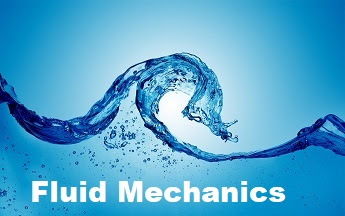## fluid mechanics handwritten notes pdf | fluid mechanics notes mechanical engineering | fluid mechanics pdf notes | fluid mechanics pdf| Mechanical Engineering Notes | Written by Ashish Sirthe branch of hydro aeromechanics that deals with the movement of compressible gaseous and liquid mediums and their interactions with solids. As a part of physics, gas dynamics is related to thermodynamics and acoustics.Thermal Engineering is a specialized sub-discipline of mechanical engineering and chemical engineering that deals with the movement of heat energy and transfer. The energy can be transformed between two mediums or transferred into other forms of energy.

## Fluid Mechanics notes Syllabus

CH-1 Fluid Static’s : Review of Basic concepts &properties of the fluid . Pressure at a point, pressure variation in static fluid, Absolute and gauge pressure, manometers, Forces on plane and curved surfaces ; buoyant force, Stability of floating and submerged bodies, Relative equilibrium.

CH-2 Kinematics of Flow : Types of flow-ideal & real , steady & unsteady, uniform & non-uniform, one, two and three dimensional flow, path lines, streak-lines, streamlines and stream tubes; continuity equation for one and three dimensional flow, rotational & irrotational flow, circulation, stagnation point, separation of flow, sources & sinks, velocity potential, stream function, flow net & its applications , method of drawing flow nets.

CH-3 Dynamics of Flow: Euler’s equation of motion along a streamline and derivation of Bernoulli’s equation, application of Bernoulli’s equation, energy correction factor, linear momentum equation for steady flow; momentum correction factor. The moment of momentum equation, forces on fixed and moving vanes and other applications. Fluid Measurements: Velocity measurement ( Pitot tube, current meters etc.); flow measurement (orifices, nozzles, mouth pieces, orifice meter, nozzle meter, venturi-meter, weirs and notches).

CH-4 Dimensional Analysis : Dimensional analysis, dimensional homogeneity, use of Buckingham-pi theorem, calculation of dimensionless numbers

CH-5 Introduction to boundary layer, Boundary layer development on a flat plate and its characteristics – Boundary layer thickness, displacement thickness, momentum thickness, energy thickness. Momentum equation for boundary layer by Von karman, drag on flat plate, boundary layer separation and its control. Aerofoil theory, lift and drag coefficients, streamlined and bluff bodies.

CH-6 Flow through Pipes : Reynolds experiment & Reynolds number, laminar & turbulent flow, Introduction to Navier Stokes’ Equation, relation between shear & pressure gradient, laminar flow through circular pipes, friction factor, laminar flow between parallel plates, hydrodynamic lubrication.

## ChAPTER-1

CH-1 Fluid Static’s : Review of Basic concepts &properties of the fluid . Pressure at a point, pressure variation in static fluid, Absolute and gauge pressure, manometers, Forces on plane and curved surfaces ; buoyant force, Stability of floating and submerged bodies, Relative equilibrium.